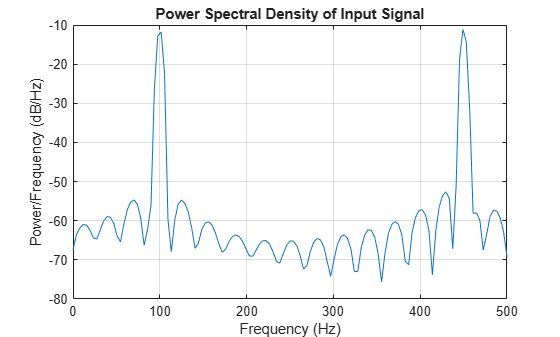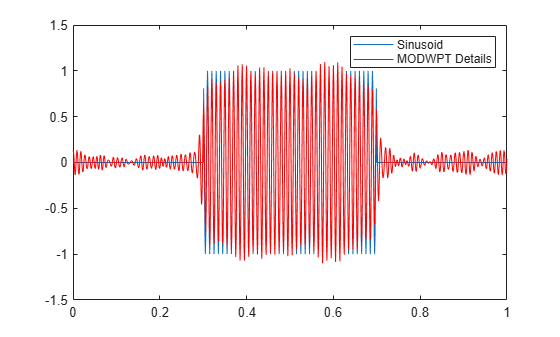# modwptdetails

Maximal overlap discrete wavelet packet transform details

## Syntax

``w = modwptdetails(x)``
``w = modwptdetails(x,wname)``
``w = modwptdetails(x,lo,hi)``
``w = modwptdetails(___,lev)``
``````[w,packetlevs] = modwptdetails(___)``````
``````[w,packetlevs,cfreq] = modwptdetails(___)``````
``[___] = modwptdetails(___,FullTree=tf)``

## Description

example

````w = modwptdetails(x)` returns the maximal overlap discrete wavelet packet transform (MODWPT) details for the 1-D signal, `x`. The MODWPT details provide zero-phase filtering of the signal. By default, `modwptdetails` returns only the terminal nodes, which are at level 4 or at level `floor(log2(numel(x)))`, whichever is smaller. NoteTo decide whether to use `modwptdetails` or `modwpt`, consider the type of data analysis you need to perform. For applications that require time alignment, such as nonparametric regression analysis, use `modwptdetails`. For applications where you want to analyze the energy levels in different packets, use `modwpt`. For more information, see Algorithms. ```

example

````w = modwptdetails(x,wname)` uses the orthogonal wavelet specified by `wname`.```

example

````w = modwptdetails(x,lo,hi)` uses the orthogonal scaling filter, `lo`, and wavelet filter, `hi`.```
````w = modwptdetails(___,lev)` returns the terminal nodes of the wavelet packet tree at positive integer level `lev`. ```

example

``````[w,packetlevs] = modwptdetails(___)``` returns a vector of transform levels corresponding to the rows of `w`. ```

example

``````[w,packetlevs,cfreq] = modwptdetails(___)``` returns `cfreq`, the center frequencies of the approximate passbands corresponding to the MODWPT details in `w`.```

example

````[___] = modwptdetails(___,FullTree=tf)`, where `tf` is `false`, returns details about only the terminal (final-level) wavelet packet nodes. If you specify `true`, then `modwptdetails` returns details about the full wavelet packet tree down to the default or specified level. The default value for `tf` is `false`.```

## Examples

collapse all

Obtain the MODWPT of an electrocardiogram (ECG) signal using the default length 18 Fejér-Korovkin (`'fk18'`) wavelet and the default level, 4.

```load wecg wptdetails = modwptdetails(wecg);```

Demonstrate that summing the MODWPT details over each sample reconstructs the signal.

```xrec = sum(wptdetails,1); max(abs(wecg-xrec'))```
```ans = 1.7903e-11 ```

Obtain the MODWPT details for a signal consisting of 100 Hz and 450 Hz sine waves. Each row of the `modwptdetails` output corresponds to a separate frequency band.

```dt = 0.001; fs = 1/dt; t = 0:dt:1; x = (sin(2*pi*100*t)+sin(2*pi*450*t)); [lo,hi] = wfilters("fk22"); wptdetails = modwptdetails(x,lo,hi);```

Use `modwpt` to obtain the energy and center frequencies of the signal. Plot the energy in the wavelet packets. The fourth and fifteenth frequency bands contain most of the energy. Other frequency bands have significantly less energy. The frequency ranges of fourth and fifteenth bands are approximately 94-125 Hz and 438-469 Hz, respectively.

```[wpt,~,cfreqs,energy] = modwpt(x,lo,hi); bar(1:16,energy); xlabel("Packet") ylabel("Packet Energy") title("Energy by Wavelet Packet")```Plot the power spectral density of the input signal.

```pwelch(x,[],[],[],fs,"onesided") title("Power Spectral Density of Input Signal")```Show that the MODWPT details have zero-phase shift from the 100 Hz input sine.

```p4 = wptdetails(4,:); tiledlayout(2,1) nexttile plot(t,sin(2*pi*100*t).*(t>0.3 & t<0.7)) title("100 Hz Sine Wave") ylabel("Amplitude") nexttile plot(t,p4.*(t>0.3 & t<0.7),"r") title("MODWPT Details") ylabel("Amplitude") xlabel("Time (s)")```Create a 100 Hz time-localized sinusoid in noise. The sampling rate is 1000 Hz. Obtain the MODWPT at level 4 using the length 22 Fejér-Korovkin (`'fk22'`) wavelet. Also obtain the center frequencies of the approximate passbands corresponding to the MODWPT details.

```Fs = 1000; t = 0:1/Fs:1; x = cos(2*pi*100*t).*(t>0.3 & t<0.7)+0.25*randn(size(t)); [wptdetails,~,cf] = modwptdetails(x,"fk22");```

The center frequencies are in cycles per sample. Convert the center frequencies to hertz.

`Fs*cf`
```ans = 16×1 15.6250 46.8750 78.1250 109.3750 140.6250 171.8750 203.1250 234.3750 265.6250 296.8750 ⋮ ```

The fourth center frequency is closest to the frequency of the sinusoid. Extract the details from the corresponding node.

`p4 = wptdetails(4,:);`

Compare the extracted details with the sinusoid. The MODWPT details represent zero-phase filtering of the input signal with an approximate passband of $\left[3Fs/{2}^{5},4Fs/{2}^{5}\right)$, where $Fs$ is the sampling frequency.

```plot(t,cos(2*pi*100*t).*(t>0.3 & t<0.7)); hold on plot(t,p4,"r") legend("Sinusoid","MODWPT Details") hold off```Obtain the MODWPT details of an ECG waveform using the length 22 Fejér-Korovkin scaling and wavelet filters.

```load wecg [lo,hi] = wfilters("fk22"); wpt = modwptdetails(wecg,lo,hi);```

Load the ECG signal, which has 2048 samples. Obtain the MODWPT details of the full wavelet packet tree for the signal. Use the default length 18 Fejér-Korovkin (`'fk18'`) wavelet.

```load wecg [w,packetlevels] = modwptdetails(wecg,FullTree=true);```

Confirm the terminal nodes of the packet tree are at level 4.

`max(packetlevels)`
```ans = 4 ```

Extract and plot the node coefficients at level 3, node 2.

```p3 = w(packetlevels==3,:); plot(p3(3,:)) axis tight title("Node (3,2) MODWPT Details")```## Input Arguments

collapse all

Input signal, specified as a row or column vector. `x` must have at least two elements.

Data Types: `single` | `double`
Complex Number Support: Yes

Analyzing wavelet, specified as a character vector or string scalar. The wavelet must be orthogonal. Orthogonal wavelets are designated as type 1 wavelets in the wavelet manager, `wavemngr`.

Valid built-in orthogonal wavelet families are: Best-localized Daubechies (`"bl"`), Beylkin (`"beyl"`), Coiflets (`"coif"`), Daubechies (`"db"`), Fejér-Korovkin (`"fk"`), Haar (`"haar"`), Han linear-phase moments (`"han"`), Morris minimum-bandwidth (`"mb"`), Symlets (`"sym"`), and Vaidyanathan (`"vaid"`).

For a list of wavelets in each family, see `wfilters`. You can also use `waveinfo` with the wavelet family short name. For example, `waveinfo("db")`. Use `wavemngr("type",wn)` to determine if the wavelet wn is orthogonal (returns 1). For example, `wavemngr("type","db6")` returns 1.

Filters, specified as a pair of even-length real-valued vectors. `lo` is the scaling (lowpass) filter and `hi` is the wavelet (highpass) filter. The filters must satisfy the conditions for an orthogonal wavelet. For more information, see `wfilters` and `isorthwfb`. You cannot specify both `wname` and a filter pair `lo,hi`.

Note

By default, the `wfilters` function returns two pairs of filters associated with an orthogonal or biorthogonal wavelet you specify. To agree with the usual convention in the implementation of `MODWPT` in numerical packages, when you specify an orthogonal wavelet `wname`, the `modwptdetails` function internally uses the second pair of filters returned by `wfilters`. For example,

```wptdetails = modwptdetails(x,"db2");```

is equivalent to

```[~,~,lo,hi] = wfilters("db2"); wptdetails = modwptdetails(x,lo,hi);```

This convention is different from the one followed by most Wavelet Toolbox™ discrete wavelet transform functions when decomposing a signal. Most functions internally use the first pair of filters.

Data Types: `single` | `double`

Transform level, specified as a positive integer less than or equal to `floor(log2(numel(x)))`.

Return tree option, specified as a numeric or logical `0` (`false`) or `1` (`true`). If `tf` is `false`, then `modwptdetails` returns details about only the terminal (final-level) wavelet packet nodes. If you specify `true`, then `modwptdetails` returns details about the full wavelet packet tree down to the default or specified level.

For the full wavelet packet tree, `w` is a 2j+1-2-by-`numel`(`x`) matrix. Each level j has 2j wavelet packet details.

## Output Arguments

collapse all

Wavelet packet tree details, returned as a matrix with each row containing the sequency-ordered wavelet packet details for the terminal nodes. The terminal nodes are at level 4 or at level `floor(log2(numel(x)))`, whichever is smaller. The MODWPT details are zero-phase-filtered projections of the signal onto the subspaces corresponding to the wavelet packet nodes. The sum of the MODWPT details over each sample reconstructs the original signal.

For the default terminal nodes, w is a 2j-by-`numel`(`x`) matrix. For the full packet table, at level j, `w` is a 2j+1-2-by-`numel`(`x`) matrix of sequency-ordered wavelet packet coefficients by level and index. The approximate passband for the nth row of `w` at level j is $\left[\frac{n-1}{{2}^{\left(j+1\right)}},\frac{n}{{2}^{\left(j+1\right)}}\right)$ cycles per sample, where n = 1,2,...,2j.

Transform levels, returned as a vector. The levels correspond to the rows of `w`. If `w` contains only the terminal level coefficients, `packetlevs` is a vector of constants equal to the terminal level. If `w` contains the full wavelet packet tree of details, `packetlevs` is a vector with 2j-1 elements for each level, j. To select all the MODWPT details at a particular level, use `packetlevs` with logical indexing.

Center frequencies of the approximate passbands in the `w` rows, returned as a vector. The center frequencies are in cycles per sample. To convert the units to cycles per unit time, multiply `cfreq` by the sampling frequency.

## Algorithms

The MODWPT details (`modwptdetails`) are the result of zero-phase filtering of the signal. The features in the MODWPT details align exactly with features in the input signal. For a given level, summing the details for each sample returns the exact original signal.

The output of the MODWPT (`modwpt`) is time delayed compared to the input signal. Most filters used to obtain the MODWPT have a nonlinear phase response, which makes compensating for the time delay difficult. All orthogonal scaling and wavelet filters have this response, except the Haar wavelet. It is possible to time align the coefficients with the signal features, but the result is an approximation, not an exact alignment with the original signal. The MODWPT partitions the energy among the wavelet packets at each level. The sum of the energy over all the packets equals the total energy of the input signal.

 Percival, Donald B., and Andrew T. Walden. Wavelet Methods for Time Series Analysis. Cambridge Series in Statistical and Probabilistic Mathematics. Cambridge ; New York: Cambridge University Press, 2000.

 Walden, A. T., and A. Contreras Cristan. “The Phase–Corrected Undecimated Discrete Wavelet Packet Transform and Its Application to Interpreting the Timing of Events.” Proceedings of the Royal Society of London. Series A: Mathematical, Physical and Engineering Sciences 454, no. 1976 (August 8, 1998): 2243–66. https://doi.org/10.1098/rspa.1998.0257.